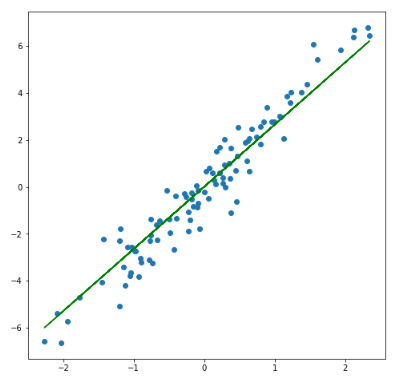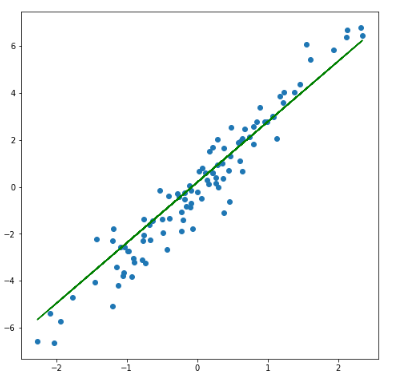# get_weights() and set_weights() functions in Keras layers

In this article, we will see the get_weights() and set_weights() functions in Keras layers. First, we will make a fully connected feed-forward neural network and perform simple linear regression. Then, we will see how to use get_weights() and set_weights() functions on each Keras layers that we create in the model. Here, I want to point out that the model shown here is of a very simple type and you can always make it more complex and powerful. Don’t worry, I will guide you around on how to do it. So, let’s begin!

### get_weights() and set_weights() in Keras

According to the official Keras documentation,

`model.layer.get_weights()`  – This function returns a list consisting of NumPy arrays. The first array gives the weights of the layer and the second array gives the biases.

`model.layer.set_weights(weights)` – This function sets the weights and biases of the layer from a list consisting of NumPy arrays with shape same as returned by `get_weights()`.

Now let us make a fully-connected neural network and perform linear regression on it. First, import all the libraries required.

```import keras
from keras.models import Sequential
from keras.layers import Dense, Activation
import numpy as np
import matplotlib.pyplot as plt```

Create a small input dataset with output targets.

```x = np.random.randn(100)
y = x*3 + np.random.randn(100)*0.8
```

Create a neural network model with 2 layers.

```model = Sequential()
model.add(Dense(4, input_dim = 1, activation = 'linear', name = 'layer_1'))
model.add(Dense(1, activation = 'linear', name = 'layer_2'))
model.compile(optimizer = 'sgd', loss = 'mse', metrics = ['mse'])```

Here, the first layer has 4 units(4 neurons/ 4 nodes), and the second layer has 1 unit.  The first layer takes the input and the second layer gives the output. The linear activation function is used as we are making a linear regression model.

get_weights()

Use the get_weights() function to get the weights and biases of the layers before training the model. These are the weights and biases with which the layers will be initialized.

```print("Weights and biases of the layers before training the model: \n")
for layer in model.layers:
print(layer.name)
print("Weights")
print("Shape: ",layer.get_weights().shape,'\n',layer.get_weights())
print("Bias")
print("Shape: ",layer.get_weights().shape,'\n',layer.get_weights(),'\n')```

Output:

```Weights and biases of the layers before training the model:

layer_1
Weights
Shape:  (1, 4)
[[ 1.0910366  1.0150502 -0.8962296 -0.3793844]]
Bias
Shape:  (4,)
[0. 0. 0. 0.]

layer_2
Weights
Shape:  (4, 1)
[[-0.74120843]
[ 0.901124  ]
[ 0.3898505 ]
[-0.36506158]]
Bias
Shape:  (1,)
[0.]```

Did you notice the shape of the weights and biases? Weights of a layer are of the shape `(input x units)` and biases are of the shape `(units,)`. `get_weights()` function returned a list consisting of Numpy arrays. Index 0 of the list has the weights array and index 1 has the bias array. The` model.add(Dense())` function has an argument `kernel_initializer` that initializes the weights matrix created by the layer. The default kernel_initializer is `glorot_uniform`. Refer to the official Keras documentation on initializers for more information on glorot_uniform and other initializers. The default initial values of biases are zero.

Fit the model and see the newly updated weights after training the model.

`model.fit(x,y, batch_size = 1, epochs = 10, shuffle = False)`
```Epoch 1/10
100/100 [==============================] - 0s 1ms/step - loss: 1.1745 - mse: 1.1745
Epoch 2/10
100/100 [==============================] - 0s 831us/step - loss: 0.6286 - mse: 0.6286
Epoch 3/10
100/100 [==============================] - 0s 823us/step - loss: 0.6275 - mse: 0.6275
Epoch 4/10
100/100 [==============================] - 0s 870us/step - loss: 0.6266 - mse: 0.6266
Epoch 5/10
100/100 [==============================] - 0s 845us/step - loss: 0.6257 - mse: 0.6257
Epoch 6/10
100/100 [==============================] - 0s 921us/step - loss: 0.6248 - mse: 0.6248
Epoch 7/10
100/100 [==============================] - 0s 1ms/step - loss: 0.6241 - mse: 0.6241
Epoch 8/10
100/100 [==============================] - 0s 843us/step - loss: 0.6234 - mse: 0.6234
Epoch 9/10
100/100 [==============================] - 0s 856us/step - loss: 0.6227 - mse: 0.6227
Epoch 10/10
100/100 [==============================] - 0s 960us/step - loss: 0.6221 - mse: 0.6221```
```print("Weights and biases of the layers after training the model: \n")
for layer in model.layers:
print(layer.name)
print("Weights")
print("Shape: ",layer.get_weights().shape,'\n',layer.get_weights())
print("Bias")
print("Shape: ",layer.get_weights().shape,'\n',layer.get_weights(),'\n')```

Output:

```Weights and biases of the layers after training the model:

layer_1
Weights
Shape:  (1, 4)
[[ 0.69193786  1.6207618  -0.7029091  -0.62313175]]
Bias
Shape:  (4,)
[-0.01838644 -0.00652554  0.01364678  0.00225105]

layer_2
Weights
Shape:  (4, 1)
[[-0.07976478]
[ 1.3966107 ]
[-0.13698433]
[-0.54812545]]
Bias
Shape:  (1,)
[0.01243665]```

Let us plot and see how well our linear line fits the model.

```plt.figure(figsize = (8,8))
plt.plot(x,y,'o',x,model.predict(x),'g')
plt.show()```It seems our model has fit well. Next, we will see the set_weights() function.

#### set_weights()

The weights passed to the set_weights() function, as mentioned earlier, must be of the same shape as returned by get_weights().

```# Setting new weights and biases
for layer in model.layers:
a,b = layer.get_weights().shape
layer.set_weights([np.random.randn(a,b), np.ones(layer.get_weights().shape)])```

This part of the code might seem confusing. Let me explain. In the line, `a,b = layer.get_weights().shape` we are extracting the shape tuple of the weights array given by get_weights() in separate variables a and b. In the last line, we pass a list of NumPy arrays – first is an array with shape (a,b) for weights and second is an array with shape corresponding to the bias array, or to say, the last line is equal to `layer.set_weights([weights_array, bias_array])`.

```print("Weights and biases of the layers after setting the new weights and biases: \n")
for layer in model.layers:
print(layer.name)
print("Weights")
print("Shape: ",layer.get_weights().shape,'\n',layer.get_weights())
print("Bias")
print("Shape: ",layer.get_weights().shape,'\n',layer.get_weights(),'\n')```

Output:

```Weights and biases of the layers after setting the new weights and biases:

layer_1
Weights
Shape:  (1, 4)
[[-0.53756154 -0.18531688  0.5337455  -0.82487863]]
Bias
Shape:  (4,)
[1. 1. 1. 1.]

layer_2
Weights
Shape:  (4, 1)
[[-1.0844678 ]
[-2.3614314 ]
[-0.36248028]
[ 0.36574388]]
Bias
Shape:  (1,)
[1.]```

Next, train the model again with the newly set weights and then see the newly updated weights after training the model.

`model.fit(x,y, batch_size = 1, epochs = 10, shuffle = False)`
```Epoch 1/10
100/100 [==============================] - 0s 922us/step - loss: 1.1968 - mse: 1.1968
Epoch 2/10
100/100 [==============================] - 0s 973us/step - loss: 0.7028 - mse: 0.7028
Epoch 3/10
100/100 [==============================] - 0s 874us/step - loss: 0.6965 - mse: 0.6965
Epoch 4/10
100/100 [==============================] - 0s 841us/step - loss: 0.6908 - mse: 0.6908
Epoch 5/10
100/100 [==============================] - 0s 877us/step - loss: 0.6858 - mse: 0.6858
Epoch 6/10
100/100 [==============================] - 0s 895us/step - loss: 0.6812 - mse: 0.6812
Epoch 7/10
100/100 [==============================] - 0s 916us/step - loss: 0.6771 - mse: 0.6771
Epoch 8/10
100/100 [==============================] - 0s 910us/step - loss: 0.6733 - mse: 0.6733
Epoch 9/10
100/100 [==============================] - 0s 866us/step - loss: 0.6699 - mse: 0.6699
Epoch 10/10
100/100 [==============================] - 0s 891us/step - loss: 0.6668 - mse: 0.6668```
```print("Weights and biases of the layers after training the model with new weights and biases: \n")
for layer in model.layers:
print(layer.name)
print("Weights")
print("Shape: ",layer.get_weights().shape,'\n',layer.get_weights())
print("Bias")
print("Shape: ",layer.get_weights().shape,'\n',layer.get_weights(),'\n')```

Output:

```Weights and biases of the layers after training the model with new weights and biases:

layer_1
Weights
Shape:  (1, 4)
[[-0.85270405 -1.0312623   0.43107903 -0.5449787 ]]
Bias
Shape:  (4,)
[0.6548792  0.3907269  0.81848514 0.95613444]

layer_2
Weights
Shape:  (4, 1)
[[-0.898052  ]
[-1.8341647 ]
[ 0.07387239]
[ 0.20433675]]
Bias
Shape:  (1,)
[1.2388276]```

Finally, plot this new model.

```plt.figure(figsize = (8,8))
plt.plot(x,y,'o',x,model.predict(x),'g')
plt.show()```Look closely at both the plots. You will notice a slight difference at the lower side of the plot. This means changing the initial weights and biases did change the output of the model. The effect of changing the initial weights might not be evident in this example with a small database and only 2 layers. To increase the complexity of the model, use a larger dataset to prevent overfitting, use more number of layers with more units in each layer, and suitable activation function.